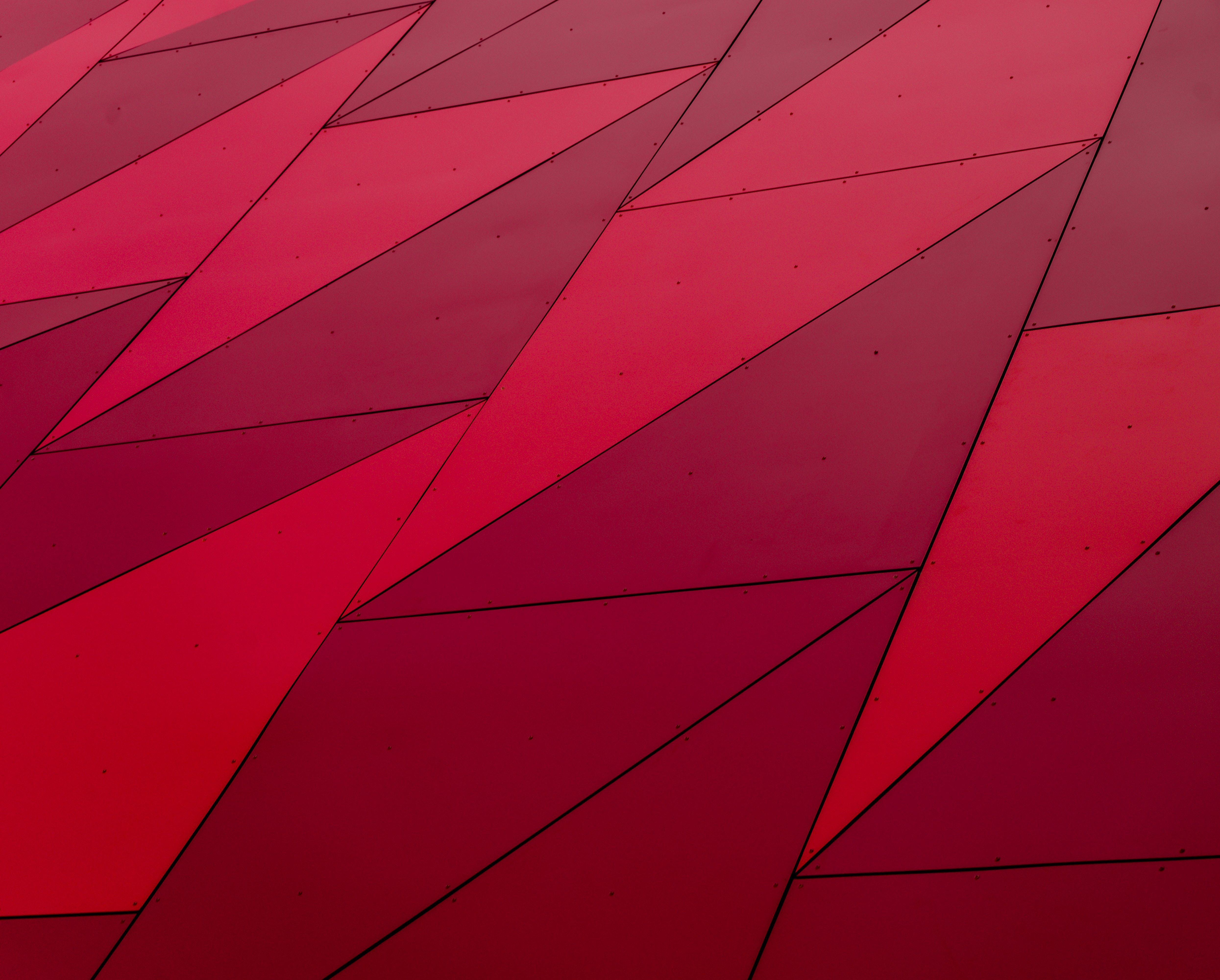# Why is Rubik Cube an abstract algebra?

Why is Rubik Cube an abstract algebra?

# Why is Rubik Cube an abstract algebra?

Find out the mathematical basis behind this popular toy

## FAQ

### What is abstract algebra?

Abstract algebra is a branch of mathematics that studies mathematical structures, such as groups, rings, and fields. It is called abstract because it deals with general concepts, which can be applied to different mathematical systems. Abstract algebra often uses symbols and operations to represent mathematical objects, such as sets and functions, and studies their properties and relationships.

### What is a Rubik’s Cube?

A Rubik’s Cube is a three-dimensional combination puzzle invented in 1974 by the Hungarian sculptor and professor of architecture Ernő Rubik. It consists of a cube with 6 faces, each made up of 9 small, colored squares, which can be rotated in different directions. The goal of the puzzle is to arrange the cube in such a way that each face has only one color.

### What makes Rubik’s Cube an abstract algebra?

The Rubik’s Cube behaves in a predictable manner which can be characterized, mathematically, as a form of algebra – abstract algebra. Abstract algebra deals with operations and structures, which can be applied to a variety of mathematical systems. The Rubik’s Cube can be modeled as a group, which is a mathematical object that has a set of elements and an operation that combines those elements in a certain way. The group theory is a branch of abstract algebra that studies groups and their properties. By examining the Rubik’s Cube from a group theoretic perspective, we can see how the cube behaves and find the optimal solutions to the puzzle.

## Why is Rubik Cube an abstract algebra?

### The group theory behind the Rubik’s Cube

The Rubik’s Cube can be modeled as a group, which is a mathematical object that consists of a set of elements and an operation that combines those elements in a certain way. The elements of the Rubik’s Cube group are the possible states of the cube, or the different arrangements of the colored squares. The operation that combines those elements is the rotation of the cube’s faces. By performing a rotation on the cube, we can transform it into a new state. The group theory studies the properties of groups, such as their order, subgroups, and homomorphisms. By examining the Rubik’s Cube group, we can analyze how the cube behaves and find the optimal solutions to the puzzle.### The mathematical structure of the Rubik’s Cube

Another aspect of abstract algebra that applies to the Rubik’s Cube is the use of structures. Abstract algebra deals with structures that consist of sets and operations, which can represent different mathematical objects, such as numbers, functions, and vectors. The Rubik’s Cube can be represented as a structure that has sets of colors and rotations, and operations that transform those sets. By analyzing the Rubik’s Cube structure, we can see how the cube behaves and find the optimal solutions to the puzzle.### The optimality of the Rubik’s Cube solutions

One of the main goals of abstract algebra is to find the best solutions to mathematical problems. The Rubik’s Cube is a prime example of such a problem, where the goal is to find the shortest sequence of rotations that will transform the cube from one state to another. By applying group theory and structure analysis to the Rubik’s Cube, we can find the optimal solutions to the puzzle, which can be expressed as algorithms that can be used to solve the cube in the fastest way possible.